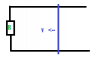# Electromagnetic induction in a U shaped conductor

• FaroukYasser

## Homework Statement

[/B]
A 0.393 m long metal bar is pulled to the left by an applied force F. The bar rides on a parallel metal rails connected through a 42.9 ohm resistor as shown in the figure. So the apparatus makes a complete circuit. You can ignore the resistance of the bars and the rails. The circuit is in an uniform 0.34 T magnetic field that is directed out of the plane of the figure. At the instant when the bar is moving to the left at 5.9 m/s. what is the rate at which the applied force is doing work on the bar?

## Homework Equations

Emf = -Blv, I = |-Blv|/R = Blv/R. F=ILB

## The Attempt at a Solution

[/B]
For my answer, I know that F=ILB but I=BLv/R so F=(B^2*L^2*v)/R. And we know that power = Force * velocity so we get: P = Fv = (BLV)^2/R. I subbed in the values and I got 14.5 mW. However, the textbook disagrees and says its 50.4 mW, where did I go wrong?

#### Attachments

•Question.PNG
468 bytes · Views: 1,180
I think your result is correct: Calculated different:

ψ = B * A , ψ is the flux , A is area.

Emf = dψ/dt = B*dA/dt = 0.34T * 0.393m * 5.9m/s.

P = Emf2 / R

The wording of the problem does not necessarily imply that the applied force equals the magnetic force (BLv). For example, the rod could have a nonzero acceleration "at the instant when the bar is moving at 5.9 m/s to the left". The sum of the applied force vector and magnetic force vector would then equal mrod ##\vec{a}## (assuming no friction between the rod and the rails).

Anyway, to get a definite answer it seems natural to make the assumption of constant speed and no friction so that the applied force equals the magnetic force. So, I think your answer is correct.